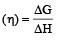Courses

# Test: Electrochemistry 2 - From Past 28 Years Questions

## 30 Questions MCQ Test Chemistry 28 Years Past year papers for NEET/AIPMT Class 12 | Test: Electrochemistry 2 - From Past 28 Years Questions

Description
This mock test of Test: Electrochemistry 2 - From Past 28 Years Questions for Class 12 helps you for every Class 12 entrance exam. This contains 30 Multiple Choice Questions for Class 12 Test: Electrochemistry 2 - From Past 28 Years Questions (mcq) to study with solutions a complete question bank. The solved questions answers in this Test: Electrochemistry 2 - From Past 28 Years Questions quiz give you a good mix of easy questions and tough questions. Class 12 students definitely take this Test: Electrochemistry 2 - From Past 28 Years Questions exercise for a better result in the exam. You can find other Test: Electrochemistry 2 - From Past 28 Years Questions extra questions, long questions & short questions for Class 12 on EduRev as well by searching above.
QUESTION: 1

### On electrolysis of dilute sulphuric acid using platinum electrodes, the product obtained at the anode will be 

Solution:

At anode :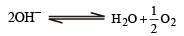QUESTION: 2

### Which of the following is an insulator ? 

Solution:

Diamond is an insulator.

QUESTION: 3

### The standard reduction poten tials at 25°C of Li+/ Li, Ba 2+ / Ba, Na+ / Na and Mg 2+ / Mg are – 3.03, – 2.73, – 2.71 and – 2.37 volt respectively. Which one of the following is the strongest oxidising agent? 

Solution:

Higher the reduction potential, stronger is the oxidizing agent.

QUESTION: 4

The most durable metal plating on iron to protect against corrosion is 

Solution:

This is because zinc has higher oxidation potential than Ni, Cu and Sn. The process of coating of iron surface with zinc is known as galvanization. Galvanized iron sheets maintain their lustrue due to the formation of protective layer of basic zinc carbonate.

QUESTION: 5

On heating one end of a piece of a metal, the other end becomes hot because of 

Solution:

When one end of a metal is heated, t he free electrons are energised and move to the other end. It heats up the other end of the metal.

QUESTION: 6

If 0.5 amp current is passed through acidified silver nitrate solution for 100 minutes. The mass of silver deposited on cathode, is (eq.wt.of silver nitrate = 108) 

Solution:

Given current (i)  = 0.5 amp;
Time (t) = 100 minutes × 60 = 6000 sec
Equivalent weight of silver nitrate (E) = 108.
According to Faraday's first law of electrolysis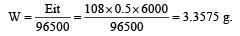QUESTION: 7

An electr och emical cell is set up as: Pt; H2 (1atm)|HCl(0.1 M) || CH3COOH (0.1 M)| H2 (1atm); Pt. The e.m.f of this cell will not be zero, because 

Solution:

For a concentration cell having different concentrations of ions.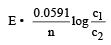If all the concentrations are identical then obviously the cell voltage is zero. But as the pH of 0.1 M HCl (strong acid) & pH of 0.1M CH3 COOH is (weak acid) not same, therefore the cell voltage is not zero.

QUESTION: 8

On passing a current of 1.0 ampere for 16 min and 5 sec through one litre solution of CuCl2, all copper of the solution was deposited at cathode. The strength of CuCl2 solution was (Molar mass of Cu= 63.5; Faraday constant = 96,500 Cmol–1) 

Solution:

By Faraday's Ist Law,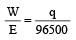(where q = it = charge of ion) we know that no of equivalent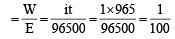(where i= 1 A, t = 16×60+5 = 965 sec.) Since, we know that

Normality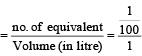= 0.01 N

QUESTION: 9

Equivalent conductances of NaCl, HCl and CH3COONa at infinite dilution are 126.45, 426.16 and 91 ohm–1 cm2 respectively. The equivalent conductance of CH3COOH at infinite dilution would be 

Solution:

By Kohlraush's law,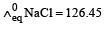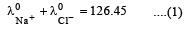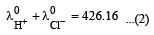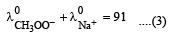on adding (2) and (3) then subtract (1) from it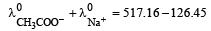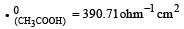QUESTION: 10

If 0.01 M solution of an electrolyte has a resistance of 40 ohms in a cell having a cell constant of 0.4 cm–1, then its molar conductance in ohm–1 cm2 mol–1 is 

Solution:

Given molarity = 0.01 M Resistance = 40 ohm;

Cell constant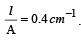Specific conductivity (k)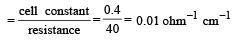Molar conductance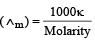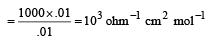QUESTION: 11

Eº for the cell,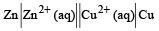1.10 V at 25ºC. The equilibrium constant for the cell reaction: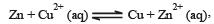Solution:

Given for the reaction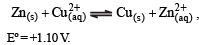At equilibrium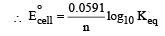here (n →  number of exchange of electrons)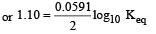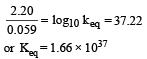QUESTION: 12

Standard potentials (Eº) for some half-reactions are given below :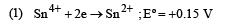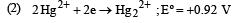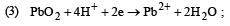Eº = +1.45 V

Based on the above, which one of the following statements is correct ? 

Solution:

In elctrochemical series, Sn is above hydrogen and Hg below hydrogen. Elements above hyrogen are reducing in nature while below hydrogen are oxidising in nature.

QUESTION: 13

For the cell reaction,  Cu2+ (C1, aq) + Zn(s) = Zn2+ (C2, aq) + Cu(s) of an electrochemical cell, the change in free energy, ΔG, at a given temperature is a function of

Solution: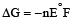For concentration cell,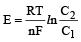In it R, T, n and F are constant So E is based upon ln C2 / C1

Now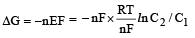= –RTlnC2/C1

At constant temperature ΔG is based upon ln C2/C1.

QUESTION: 14

Without losing its concentration ZnCl2 solution cannot be kept in contact with 

Solution:

With out losing its concentrati on Zn Cl2 solution can not kept in contact with Al because Al is more reactive than Zn due to electrode (reduction) potential.

QUESTION: 15

What is the Eºcell for the reaction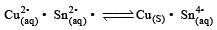at 25ºC if the equilibrium constant for the reaction is  1 × 106? 

Solution: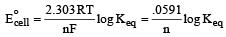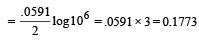QUESTION: 16

Th e ionic conductan ce of Ba2+ an d Cl are respectively 127 and 76 ohm–1 cm2 at infinite dilution. The equivalent conductance (in ohm–1 cm2) of BaCl2 at infinite dilution will be : 

Solution:

The equivalent con ductance of BaCl2 at infinite dilution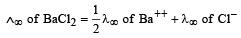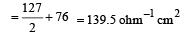QUESTION: 17

Cu +aq is unstable in solution and undergoes simultaneous oxidation and reduction according to the reaction :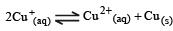choose correct Eº for above reaction if Eº Cu2+/Cu = 0.34 V and Eº Cu2+/Cu+ = 0.15 V

Solution: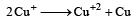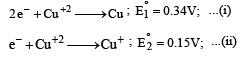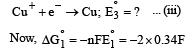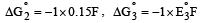Again,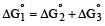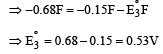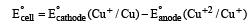= 0.53 – 0.15 = 0.38 V.

QUESTION: 18

Specific conductance of a 0.1 N KCl solution at 23ºC is 0.012 ohm–1 cm–1. Resistance of cell containing the solution at same temperature was found to be 55 ohm. The cell constant is 

Solution:

Given specific conductance of the solution (k) = 0.012 ohm–1 cm–1 and resistance (R) = 55 ohm. We know that cell constant = Specific conductance × Observed resistance = 0.012 × 55= 0.66cm–1.

QUESTION: 19

Standard electrode potentials are : Fe+2/Fe [ Eº = –0.44]; Fe+3/Fe+2  Eº = + 0.77 ; If Fe+2, Fe+3 and Fe blocks are kept together, then 

Solution: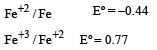The metals having higher negative electrode potential can displaced metals having lower values of negative electrode potential from their salt solutions.

Hence B is correct.

QUESTION: 20

The most convenient method to protect the bottom of ship made of iron is 

Solution:

For bottom of ship to be protected it is connected with more reactive metal than iron like magnesium. This technique is called cathodic protection.

QUESTION: 21

In the silver plating of copper, K[Ag(CN)2] is used instead of AgNO3. The reason is 

Solution:

In the silver plating of copper, K[Ag(CN)2] is used instead of AgNO3. Copper being more electropositive readily precipitate silver from their salt solution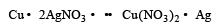whereas in K[Ag (CN)2] solution a complex anion [Ag(CN)2] is formed and hence Ag+ are less available in the solution and therefore copper cannot displace Ag from its  complex ion.

QUESTION: 22

Which reaction is not feasible? 

Solution: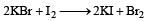reaction is not possible because Br ion is not oxidised in Br2 with I2 due to higher electrode (oxidation) potential of I2 than bromine.

QUESTION: 23

In electrolysis of NaCl when Pt electrode is taken then H2 is liberated at cathode while with Hg cathode it forms sodium amalgam. This is because 

Solution:

In electrolysis of NaCl when Pt electrode is taken then H2 liberated at cathode while with Hg cathode it forms sodium amalgam because more voltage is required to reduce H+ at Hg than Pt.z

QUESTION: 24

The e.m.f. of a Daniell cell at 298 K is E1.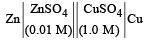When the concentration of ZnSO4 is 1.0 M and that of CuSO4 is 0.01 M, the e.m.f. changed to E2.What is the relationship between E1 and E2? 

Solution:

Cell reaction is,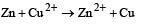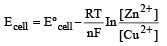Greater the factor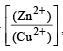less is the EMF

Hence E1 > E2

QUESTION: 25

On the basis of the information available from the reaction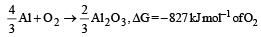the minimum e.m.f required to carry out an electrolysis of Al2O3 is (F = 96500 C mol–1)

Solution:

ΔG = –nEF

For 1 mol of Al, n = 3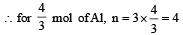According to question, 827 x1000 = 4 x E x 96500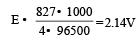QUESTION: 26

The standard e.m.f. of a galvanic cell involving cell reaction with n = 2 is found to be 0.295 V at 25°C. The equilibrium constant of the reaction would be    (Given F = 96500 C mol–1; R = 8.314JK– 1mol–1)

Solution: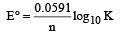Here, n = 2, E° = 0.295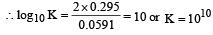QUESTION: 27

4.5 g of aluminium (at. mass 27 amu) is deposited at cathode from Al3+ solution by a certain quantity of electric charge. The volume of hydrogen produced at STP from H+ ions in solution by the same quantity of electric charge will be 

Solution:

No. of gram equivalent of H+ = No. of gm eq. of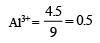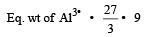No. of gm eq. of H+ = no. of mole of H+ Hence Mass of H+ = 0.5 × 1g = 0.5 g

We know that, 2g H2 at STP = 22.4 L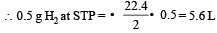QUESTION: 28

If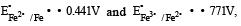, the standard EMF of the reaction Fe + 2Fe3+ → 3Fe2+  will be 

Solution: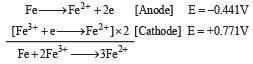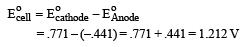QUESTION: 29

A hypoth etical electrochemical cell is shown below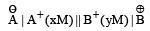The emf measured is +0.20 V. The cell reaction is

Solution:

The cell reacton is as follows :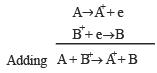Efficiency of a fuel cell